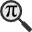## General

Display information for equation id:math.224934.5 on revision:224934

* Page found: Interest rate (eq math.224934.5)

(force rerendering)

Cannot find the equation data in the database. Fetching from revision text.

Occurrences on the following pages:

Hash: f570e1cf266d0ace43989bc03f945bc0

TeX (original user input):

p_e\,\!


TeX (checked):

p_{e}\,\!


### LaTeXML (experimental; uses MathML) rendering

MathML (826 B / 327 B) :$p_{e}\,\!$
<math xmlns="http://www.w3.org/1998/Math/MathML" id="p1.1.m1.1" class="ltx_Math" alttext="{\displaystyle p_{e}\,\!}" display="inline">
<semantics id="p1.1.m1.1a">
<msub id="p1.1.m1.1.5" xref="p1.1.m1.1.5.cmml">
<mi id="p1.1.m1.1.1" xref="p1.1.m1.1.1.cmml">p</mi>
<mi id="p1.1.m1.1.2.1" xref="p1.1.m1.1.2.1.cmml">e</mi>
</msub>
<annotation-xml encoding="MathML-Content" id="p1.1.m1.1b">
<apply id="p1.1.m1.1.5.cmml" xref="p1.1.m1.1.5">
<csymbol cd="ambiguous" id="p1.1.m1.1.5.1.cmml">subscript</csymbol>
<ci id="p1.1.m1.1.1.cmml" xref="p1.1.m1.1.1">p</ci>
<ci id="p1.1.m1.1.2.1.cmml" xref="p1.1.m1.1.2.1">e</ci>
</apply>
</annotation-xml>
<annotation encoding="application/x-tex" id="p1.1.m1.1c">{\displaystyle p_{e}\,\!}</annotation>
</semantics>
[/itex]


SVG image empty. Force Re-Rendering

SVG (2.021 KB / 1.051 KB) :

### MathML with SVG or PNG fallback (recommended for modern browsers and accessibility tools) rendering

SVG image empty. Force Re-Rendering

SVG (0 B / 8 B) :

PNG (0 B / 8 B) :

$p_{e}\,\!$## Translations to Computer Algebra Systems

### Translation to Maple

In Maple: p[e]

e: You use a typical letter for a constant [the mathematical constant e == Napier's constant == 2.71828182845...].

We keep it like it is! But you should know that Maple uses exp(1) for this constant.

If you want to translate it as a constant, use the corresponding DLMF macro \expe

### Translation to Mathematica

In Mathematica: Subscript[p, e]

e: You use a typical letter for a constant [the mathematical constant e == Napier's constant == 2.71828182845...].

We keep it like it is! But you should know that Mathematica uses E for this constant.

If you want to translate it as a constant, use the corresponding DLMF macro \expe

## Similar pages

Calculated based on the variables occurring on the entire Interest rate page

## Identifiers

• $p_{e}$### MathML observations

0results

0results

no statistics present please run the maintenance script ExtractFeatures.php

0 results

0 results